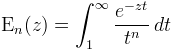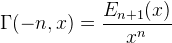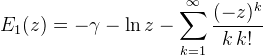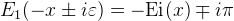Computes the generalized exponential integral.

Namespace:  Meta.Numerics.Functions
Assembly:  Meta.Numerics (in Meta.Numerics.dll) Version: 4.1.4Syntax
```public static double IntegralE(
int n,
double x
)```

#### Parameters

n
Type: SystemInt32
The order parameter.
x
Type: SystemDouble
The argument, which must be non-negative.

#### Return Value

Type: Double
The value of En(x).Exceptions
ExceptionCondition
ArgumentOutOfRangeExceptionx is negative.Remarks

The generalized exponential integral is defined as:It is related to the incomplete Gamma function (Gamma(Double, Double)) for negative, integer shape parameters.For n=1, it expressible as a simple power series.For negative x, E1(x) develops an imaginary part, but its real part is given by the Ei(x) function (IntegralEi(Double)).To compute E1(z) in the entire complex plane, use Ein(Complex).

Sometimes the function E1(z) is called the exponential integral, and sometimes that name is used for Ei(x). In hydrology, E1(x) is sometimes called the Well function.See Also